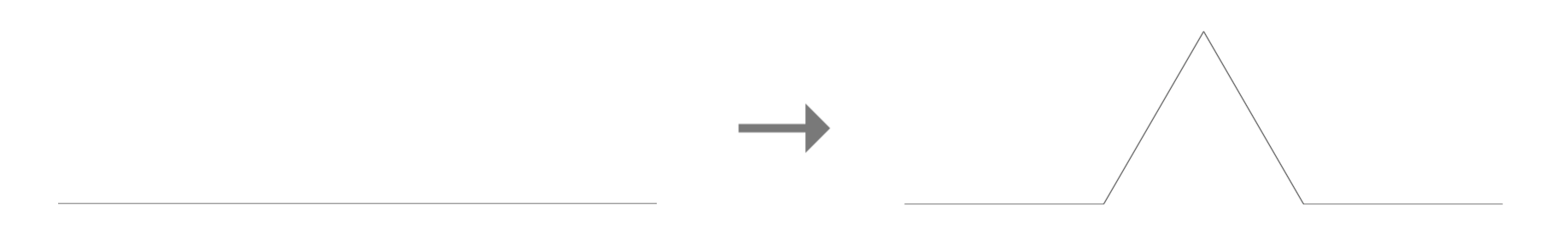Koch Snowflake Area

The Koch Snowflake has an infinite perimeter, but all its squiggles stay crumpled up in a finite area. So how big is this finite area, exactly?

To answer that, let’s look again at The Rule.When we apply The Rule, the area of the snowflake increases by that little triangle under the zigzag. So we need two pieces of information:

1. Each time we apply The Rule, how many little triangles do we add?
2. Each time we apply The Rule, what’s the size of each little triangle we’re adding?

Let’s tackle these one at a time.

1) How many little triangles do we add? This part’s pretty simple. We start with a triangle, which means we have $3$ line segments to work with. For each line segment, we add $1$ triangle, and our line segment becomes $4$ little segments. So we end up with $3$ new triangles and $3 \cdot 4$ total line segments. Then when we apply The Rule a second time, we take our $3 \cdot 4$ line segments and add a triangle for each one, finishing with $3 \cdot 4 \cdot 4$ little line segments. Then the next time we apply The Rule, we add $3 \cdot 4 \cdot 4$ new little triangles and end up with $3 \cdot 4 \cdot 4 \cdot 4$ tiny line segments. In general, when we apply The Rule for the $n$th time, we add $3 \cdot 4^{n-1}$ new triangles.

2) What’s the size of each little triangle we’re adding? This one’s a little trickier. Let’s start with side lengths. Suppose we say our original triangle has side length $s$. When we apply The Rule to the triangle, the new little triangles we’re adding on have side length $\frac{s}{3}$. (Look again at our Rule if that doesn’t make sense.) The lengths of all our line segments are now $\frac{s}{3}$, so when we apply The Rule a second time, the side lengths of the new little triangles are $\frac{s}{3 \cdot 3}$. Then when we apply The Rule a third time, the new side lengths are $\frac{s}{3 \cdot 3 \cdot 3}$. So in general, when we apply The Rule for the $n$th time, the new little triangles have side length $\frac{s}{3^n}$. Now let’s convert all this into triangle areas. There’s a formula for the area of an equilateral triangle with side length $s$: $s^2 \cdot \frac{\sqrt{3}}{4}$. (See if you can figure out why!) Now when we apply The Rule to the triangle, our new little triangles that we’re adding to the area have side length $\frac{s}{3}$, so we substitute that into the formula and get $\frac{s^2}{3^2} \cdot \frac{\sqrt{3}}{4}$. When we apply The Rule a second time, the side length of the new little triangles is $\frac{s}{3^2}$, so the area becomes $\frac{s^2}{(3^2)^2} \cdot \frac{\sqrt{3}}{4}$. Then when we apply The Rule a third time, the triangle side length is $\frac{s}{3^3}$, so the area becomes $\frac{s^2}{(3^3)^2} \cdot \frac{\sqrt{3}}{4}$. In general, when we apply The Rule for the $n$th time, the new little triangles have side length $\frac{s}{3^n}$ and area $\frac{s^2}{(3^n)^2} \cdot \frac{\sqrt{3}}{4}$.

Okay, let’s put this all together. When we apply The Rule for the $n$th time, we add $3 \cdot 4^{n-1}$ new triangles, each with area $\frac{s^2}{(3^n)^2} \cdot \frac{\sqrt{3}}{4}$. So the total area that we’re adding to the snowflake when we apply The Rule for the $n$th time is

$$3 \cdot 4^{n-1} \cdot \dfrac{s^2}{(3^n)^2} \cdot \dfrac{\sqrt{3}}{4}$$

$$= \dfrac{s^2\sqrt{3} \cdot 4^{n-2}}{3^{2n-1}}$$

Yikes.

In order to finish up this calculation and figure out the area of the snowflake, we need to use this expression to add up all the triangle areas that make up the entire snowflake. In other words, we need to add the area of our original triangle, the total area we get from applying The Rule once, the total area we get from applying The Rule a second time, the total area we get from applying The Rule a third time, and so on to infinity. That’s

$$\dfrac{s^2\sqrt{3}}{4} + \dfrac{s^2\sqrt{3}}{3 \cdot 4} + \dfrac{s^2\sqrt{3}}{3^3} + \dfrac{s^2\sqrt{3} \cdot 4}{3^5} + \dfrac{s^2\sqrt{3} \cdot 4^2}{3^7} + \ldots$$

We need to get rid of the “$\ldots$” before we can compress this into our final answer. To do this, we notice that each new term is formed by multiplying the last one by $\frac{4}{9}$. The one exception is going from the first term to the second term – we’ll need to treat the first term separately, but we’ll deal with that in a minute. There’s a formula that can help us with the “$\ldots$": when you have an infinite sum where you get the next term by multiplying the previous one by a number less than 1, the sum is equal to

$$\dfrac{a}{1-r}$$

where $a$ is the first term in the series and $r$ is the common ratio. In this case, we have to deal with the first term in our sum not following the same pattern as the rest of the terms, so we let the second term be $a$; we plug $\frac{s^2\sqrt{3}}{3 \cdot 4}$ and $\frac{4}{9}$ into the above formula, which reduces our infinite sum down to

$$\dfrac{s^2\sqrt{3}}{4} + \dfrac{\frac{s^2\sqrt{3}}{3 \cdot 4}}{1 - \frac{4}{9}}$$

$$= \dfrac{s^2\sqrt{3}}{4} + \dfrac{s^2\sqrt{3}}{3 \cdot 4} \cdot \dfrac{9}{5}$$

$$= \dfrac{s^2\sqrt{3}}{4} + \dfrac{3s^2\sqrt{3}}{20}$$

$$= \dfrac{5s^2\sqrt{3} + 3s^2\sqrt{3}}{20}$$

$$= \dfrac{8s^2\sqrt{3}}{20}$$

$$= \dfrac{2s^2\sqrt{3}}{5}$$

Whew.

That’s our answer. Whatever we set the side length of the original equilateral triangle to be, we can use this formula to find the area of the finished snowflake. We might be able to get a better idea of what this formula is telling us if we let the area of the original triangle be $a$, which we already mentioned is equal to $\frac{s^2\sqrt{3}}{4}$, and substitute that into the formula:

$$\dfrac{2s^2\sqrt{3}}{5}$$

$$= \dfrac{8 \cdot \frac{s^2\sqrt{3}}{4}}{5}$$

$$= \dfrac{8 \cdot a}{5}$$

This tells us that the area of the snowflake is $\frac{8}{5}$ times the area of the triangle we grew it from. Cool, eh?